Published: 21 October 2017

# Experimental approach for optical registration of circular time-averaged moiré images

Guangqing Lu1
Sandra Aleksiene3
Wenbin Peng4
1, 4School of Electrical and Information Engineering of Jinan University, 206 Qianshan Road, Zhuhai, 519070, Guangdong, P. R. China
2Department of Printing Machines, Vilnius Gediminas Technical University, J. Basanaviciaus 28-129, Vilnius, LT-03224, Lithuania
3Department of Mathematical Modelling, Kaunas University of Technology, Studentu 50-146, Kaunas, LT-51368, Lithuania
Corresponding Author:
Guangqing Lu
Views 23

#### Abstract

This paper presents the development of an experimental stand for the registration of time-averaged geometric circular moiré images. Optical interpretation of patterns of time-averaged moiré fringes allows to identify the parameters of circularly vibrating structures. The developed scheme has a strong potential for designing experimental schemes capable to measure angular vibrations of rotating shafts in different mechanisms, and do not require any mountable sensors.

## 1. Introduction

Optical geometric moiré is a well-developed experimental technique with a multitude of practical applications and implementations . It is well known that geometric moiré can be used not only for the determination of deformations at the centerlines of moiré fringes – it can be also used for the synthesis of the required moiré patterns [2, 3]. The same holds true for time-averaged geometric moiré [4, 5]. However, the applicability of time-averaged geometric moiré for oscillating structures in angular direction is much more complicated than for uni-directional vibrations . The main objective of this paper is to demonstrate the experimental optical setup which could be applicable for the registration of time-averaged moiré fringes generated by angularly oscillating mechanical structures.

## 2. Optical background

One-dimensional moiré grating can be represented by a harmonic function varying in interval [0; 1]:

1
$M\left(x\right)=0.5+0.5\mathrm{sin}\left(\frac{2\pi }{\lambda }x\right),$

where $x$ is the longitudinal coordinate; $\lambda$ is the pitch of the grating; 0 stands for the black color; 1 – for the white color. Harmonic vibrations around the state of equilibrium:

2
$u\left(y,t\right)=a\left(y\right)\mathrm{s}\mathrm{i}\mathrm{n}\left(\omega t+\varphi \right),$

yield time-averaged moiré fringes in the time averaged image [1, 6]:

3
$\stackrel{-}{M}\left(x,y\right)=0.5+0.5\mathrm{cos}\left(\frac{2\pi }{\lambda }x\right){J}_{0}\left(\frac{2\pi }{\lambda }a\left(y\right)\right),$

where $\omega$ is the angular frequency; $\varphi$ is the phase of harmonic oscillations; $t$ is time; and ${J}_{0}$ is the zero order Bessel function of the first kind. Time-averaged moiré fringes do form when the amplitude of harmonic oscillations $a\left(y\right)$, the pitch of the moiré grating, and the roots of the Bessel function ${r}_{k}$ are linked by the following relationship [1, 6]:

4
$\frac{2\pi }{\lambda }a\left(y\right)={r}_{k},$

where $k$ is the number of the root of ${J}_{0}$. The situation becomes much more complex when the stationary moiré grating is comprised not from an array of parallel lines – but an array of concentric circles around the fixed central point.

## 3. The experimental setup for the registration of time-averaged angular oscillations

Let us consider a basic problem for the optical determination of the angular rotation of the deformable rotating lithographic press rubber roller train (Fig. 1). The array of concentric moiré circles is well applicable for the registration of dynamic deformations of the rotating rubber cylinder – no interpretable image would be registered in the time-averaged mode otherwise. However, such experimental setup cannot interpret angular oscillations around a steady angular rotation.

Fig. 1Angular rotation of a lithographic press rubber roller train in time-averaged mode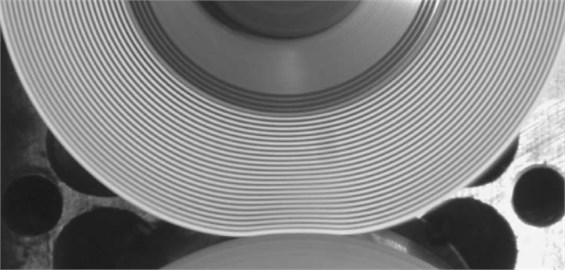A new approach is required to overcome such a problem which limits direct optical registration of angular oscillations. The natural approach is to design the cover image comprised not from an array of circular lines – but from a circular array of one-dimensional moiré gratings.

Fig. 2The general view of the experimental setup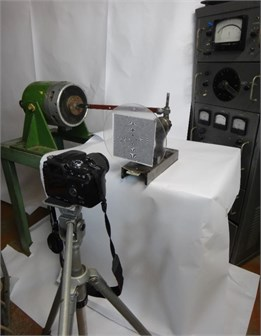Fig. 3A schematic diagram illustrating the experimental setup: low frequency generator (1), electromagnetic shaker table (2), solid foundation (2a), the cover image attached to the planar crankshaft mechanism (3), DSLR camera (4), and computer (5). See the text for a detailed description of the components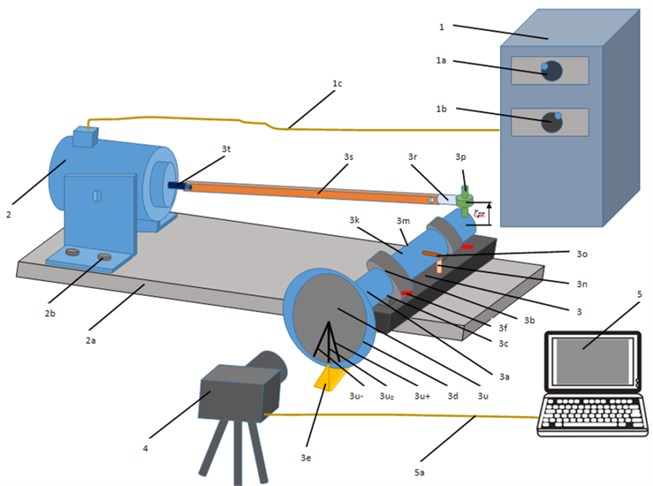A low frequency generator (1) is driving the electromagnetic shaker table (2), which in its turn is attached to the planar crankshaft mechanism (3) (Fig. 2). A DSLR camera (4) is used to register the time-averaged image. The harmonic signal from the low frequency generator can be controlled by the amplitude handle (1a) and the frequency handle (1b). The shaker table is attached to the solid metal foundation (2a); while the planar crankshaft mechanism (3) is directly fixed to the same foundation. The planar crankshaft mechanism is driven by the metal rod (3e) with joints (3d) and (3f). The longitudinal core axis of the shaker table is aligned to the axis of metal rod (3e) and is perpendicular to the longitudinal axis of the crankshaft (3a). The rotation angle of the cover image can be mechanically adjusted by changing the vertical position of joint (3f) (Fig. 3).

Fig. 4a) The static cover image comprised by an array of circular moiré gratings and b) the time averaged image of the cover image performing time-averaged angular oscillations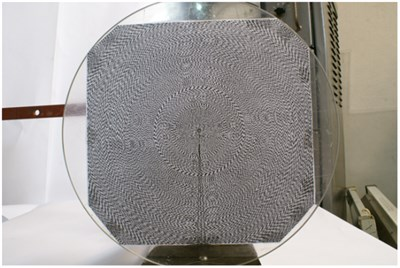a)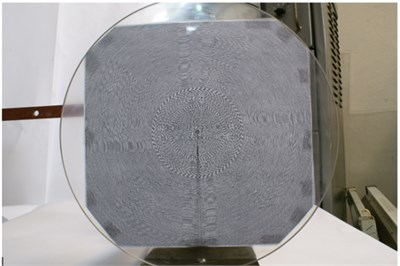b)

The ability to control the frequency and the amplitude of angular harmonic oscillations opens a possibility for experimental validation of numerical simulations. The features of the experimentally reconstructed time-averaged image of an angularly oscillating cover image are clearly illustrated in Fig. 4. Such an experimental setup.

## 4. Conclusions

The proposed experimental technique for optical registration and measurement of angular oscillations is based on the formation of a pattern of fringes in time-averaged image of circular moiré gratings. The presented measurement scheme is tested and validated for a simplified oscillating structure. However, the potential of the proposed system can be truly unleashed if it is applied for the measurement of rotating and simultaneously vibrating circular structures – what is a definite objective of future research.

#### Cited by

The 2021 flexible and printed electronics roadmap

07 September 2017
Accepted
04 October 2017
Published
21 October 2017
SUBJECTS
Measurements in engineering
Keywords
geometric moiré
angular vibrations
time-averaged image
Acknowledgements

Financial supports from the National Natural Science Foundation of China under Project No. 51475211 is acknowledged.